# Graphing Absolute Value EquationsPage 1

#### WATCH ALL SLIDES

Slide 1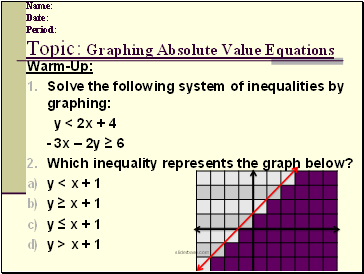## Topic: Graphing Absolute Value Equations

Name: Date: Period:

Warm-Up:

Solve the following system of inequalities by graphing:

y < 2x + 4

- 3x – 2y ≥ 6

Which inequality represents the graph below?

y < x + 1

y ≥ x + 1

y ≤ x + 1

y > x + 1

Slide 2Home-Learning Assignment #5 Review

Slide 3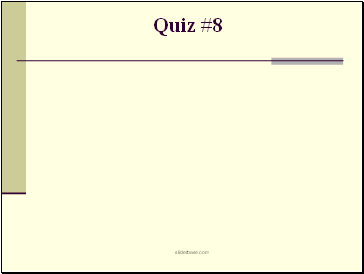Quiz #8

Slide 4## Graphing Absolute Value Equations

How do I make one of those V graphs?

Slide 5## Absolute value transformation

Vocabulary:

Translation: shifts the parent function graph horizontally and vertically.

Reflection: it creates a mirror image of the parent-function graph across the line of reflection.

Slide 6Parent Function y = │x │

Slide 7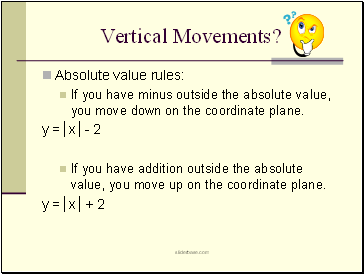## Vertical Movements?

Absolute value rules:

If you have minus outside the absolute value, you move down on the coordinate plane.

y =│x│- 2

If you have addition outside the absolute value, you move up on the coordinate plane.

y =│x│+ 2

Slide 8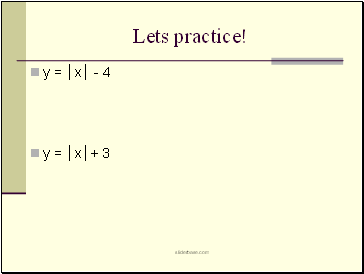Lets practice!

y = │x│ - 4

y = │x│+ 3

Slide 9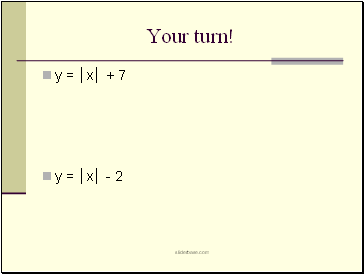y = │x│ + 7

y = │x│ - 2

Slide 10## Horizontal Movements?

Absolute value special rules:

If you have minus inside the absolute value, you move to the right on the coordinate plane.

y =│x - 2 │

If you have addition inside the absolute value, you move to the left on the coordinate plane.

y =│x + 2 │

Slide 11Lets Practice!

y =│x - 5│

y =│x + 6│

Slide 12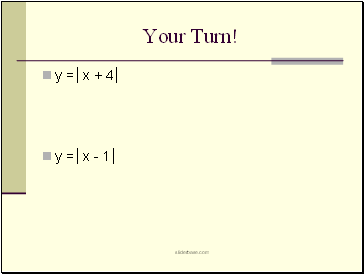y =│x + 4│

y =│x - 1│

Slide 13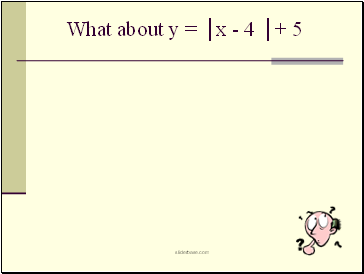What about y = │x - 4 │+ 5

Slide 14

Go to page:
1  2